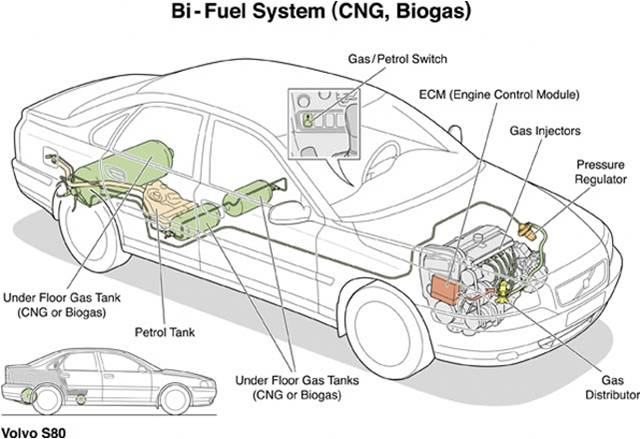# Compressed Natural Gas

$$\newcommand{\vecs}{\overset { \rightharpoonup} {\mathbf{#1}} }$$ $$\newcommand{\vecd}{\overset{-\!-\!\rightharpoonup}{\vphantom{a}\smash {#1}}}$$$$\newcommand{\id}{\mathrm{id}}$$ $$\newcommand{\Span}{\mathrm{span}}$$ $$\newcommand{\kernel}{\mathrm{null}\,}$$ $$\newcommand{\range}{\mathrm{range}\,}$$ $$\newcommand{\RealPart}{\mathrm{Re}}$$ $$\newcommand{\ImaginaryPart}{\mathrm{Im}}$$ $$\newcommand{\Argument}{\mathrm{Arg}}$$ $$\newcommand{\norm}{\| #1 \|}$$ $$\newcommand{\inner}{\langle #1, #2 \rangle}$$ $$\newcommand{\Span}{\mathrm{span}}$$ $$\newcommand{\id}{\mathrm{id}}$$ $$\newcommand{\Span}{\mathrm{span}}$$ $$\newcommand{\kernel}{\mathrm{null}\,}$$ $$\newcommand{\range}{\mathrm{range}\,}$$ $$\newcommand{\RealPart}{\mathrm{Re}}$$ $$\newcommand{\ImaginaryPart}{\mathrm{Im}}$$ $$\newcommand{\Argument}{\mathrm{Arg}}$$ $$\newcommand{\norm}{\| #1 \|}$$ $$\newcommand{\inner}{\langle #1, #2 \rangle}$$ $$\newcommand{\Span}{\mathrm{span}}$$

## Compressed Natural Gas

For years now, the developed world has used natural gas as a fuel. It is composed mostly of methane, but also composed of ethane and propane in smaller quantities. Most frequently, this fuel is moved to domestic consumers via pipelines from its source.1 In terms of usage, natural gas is experiencing faster growth compared to any other fossil fuel.3 Consumption is often from cooking or heating, and for some, utility usage for electricity instead of coal or oil.1 With the amount of energy that natural gas yields during combustion, it is a great fuel.

$\ce{CH4 (g) + 2O2 (g) -> CO2 (g) + 2H2O (l)}\nonumber$

∆H = -890 kJ/molFigure $$\PageIndex{1}$$. The transportation of CNG.Figure $$\PageIndex{2}$$. CNG above ground pipelines.

Compressed natural gas (CNG) is natural gas that has been put under a great amount of pressure.1 This occurs by pushing the gas into a smaller volume. This is due to a property of all gasses called compressibility. This property was first described by the observations of Robert Boyle. Boyle noticed that the volume of a given amount of gas at a given temperature is inversely proportional to the pressure applied by the gas. We now know this association as Boyle’s law (click here for an animation).4

V α 1/P where α means “proportional to” and n and T are constant.3

Compressed natural gas is used to power some vehicles. However, there is a high cost associated with converting a gasoline engine to run off of natural gas as the fuel, so the utilization of CNG is currently limited to vehicles such as commercial truck and taxis. To allow for reasonable storage, the CNG must be kept at a very high pressure which results in heavy fuel tanks due the requirement of thick walls. In addition, the driving range (prior to refilling) of CNG vehicles is most often significantly shorter than that of gasoline-powered vehicles so that the weight and size of the fuel tank is reasonable.1Figure $$\PageIndex{3}$$. Volvo S80 2.4-liter vehicle that has a five cylinder bi-fuel engine. The engine uses separate fuel systems as it runs primarily on compressed natural gas (biogas) but has gasoline system for back-up.

In order for natural gas to remain in a gaseous state, the pressure of the gas must remain between 1,200 and 4,000 psi. When the gas is then compressed, it reduces the volume by about 200 fold (depending on the operating pressure and temperature).3

The cost of compressed natural gas is considerably less than that of gasoline. CNG can be as inexpensive as .85 cents a gas gallon equivalent (gge) and averages one third of the price of gasoline. CNG is cleaner and therefore more environmentally-friendly than petroleum-based fuels, producing 70% less carbon monoxide, 87% less non-methane organic gas, 87% less nitrogen oxide and 20% less carbon dioxide. Although CNG results in methane production, this is offset by the significant reduction in carbon dioxide emissions.2

Example $$\PageIndex{1}$$: Volume of Methane in Tanks

Vehicle tanks of compressed natural gas can range in size from 4.7 gas gallon equivalent (gge) to 28.8 gge. They are stored at 3,600 psi at 70°F. At atmospheric pressure (14.696 psi), what volume would be of methane would you have with both of these sized tanks?

Solution:

$\text{P}_1 \text{V}_1 = \text{P}_2 \text{V}_2\nonumber$

$\text{3600} \times \text{4.7} = \text{14.696} \times \text{V}_2\nonumber$

$\text{V}_2 = \text{1151.3 gge}\nonumber$

$\text{P}_1 \text{V}_1 = \text{P}_2 \text{V}_2\nonumber$

$\text{3600} \times \text{28.8} = \text{14.696} \times \text{V}_2\nonumber$

$\text{V}_2 = \text{7055.0 gge}\nonumber$Figure $$\PageIndex{4}$$. CNG tanks in vehicle

From ChemPRIME: 9.6: Boyle&#39;s Law

References

1. Baird, C., & Cann, M. (Eds.). (2005). Environmental chemistry (3rd Edition ed.). New York: W. H. Freeman and Company.

2. Compressed Natural Gas United. http://cngunited.com/

3. Economides, M., & Mokhatab, S. (2007). Compressed natural gas: Monetizing stranded gas. Energy Tribune,

4. Kotz, J., Treichel, P., & Harman, P. (Eds.). (2003). Chemistry & chemical reactivity (5th Edition ed.). United States of America: Thomson Learning Inc.

Photos: